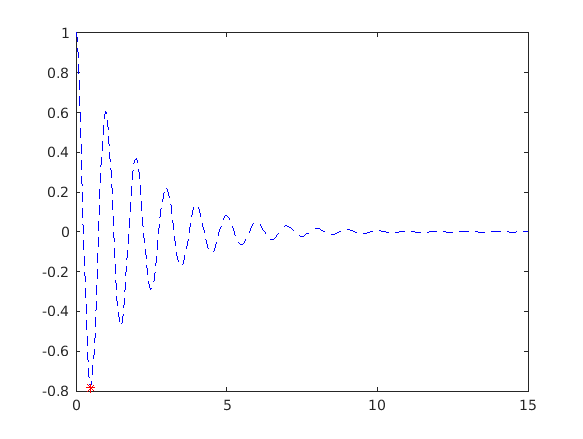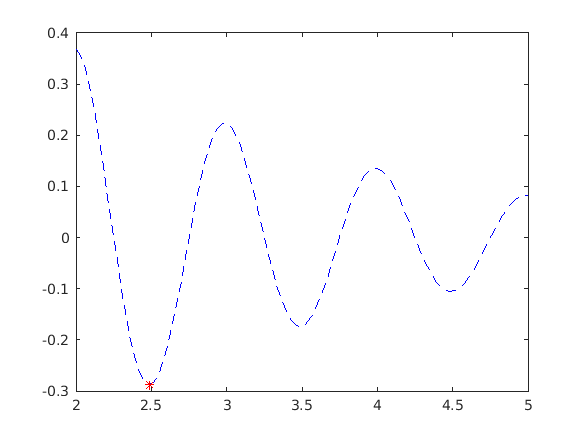Cody

# Problem 44934. Plot Damped Sinusoid

Solution 1981825

Submitted on 18 Oct 2019 by Andrew Hines
This solution is locked. To view this solution, you need to provide a solution of the same size or smaller.

### Test Suite

Test Status Code Input and Output
1   Pass
clf; t = linspace(0,15,400); y = exp(-0.5*t).*cos(2*pi.*t); m = plot_cos(y, t); f = gcf; assert(isequal([f.Children.Children.Color], [1 0 0 0 0 1])) assert(strcmp([f.Children.Children.LineStyle], 'none--')) assert(strcmp([f.Children.Children.Marker],'*none')) assert(abs(m - (-0.781239288889930)) <= 1e-4) assert(isequal([f.Children.Children.YData],[m, y]))

``
2   Pass
clf; t = linspace(2,5,100); y = exp(-0.5*t).*cos(2*pi.*t); m = plot_cos(y, t); f = gcf; assert(isequal([f.Children.Children.Color], [1 0 0 0 0 1])) assert(strcmp([f.Children.Children.LineStyle], 'none--')) assert(strcmp([f.Children.Children.Marker],'*none')) assert(abs(m - (-0.287376348726584)) <= 1e-4) assert(isequal([f.Children.Children.YData],[m, y]))

``